# Local homology

(diff) ← Older revision | Latest revision (diff) | Newer revision → (diff)

The homology groups (cf. Homology group)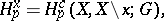defined at points, where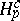is homology with compact support. These groups coincide with the direct limits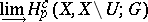over open neighbourhoodsof, and for homologically locally connectedthey also coincide with the inverse limitsThe homological dimension of a finite-dimensional metrizable locally compact spaceover(cf. Homological dimension of a space) coincides with the largest value offor which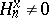, and the set of such pointshas dimension.

Let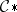be the differential sheaf overdefined by associating with each open set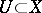the chain complex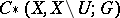. The groups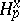are the fibres of the derived sheaves. For generalized manifolds,for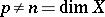. In this case the homology sequence of the pair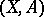with coefficients incoincides with the cohomology of the pair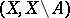with coefficients in the sheaf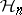(Poincaré–Lefschetz duality). The similar facts for the local cohomology of locally compact spaces do not hold.

How to Cite This Entry:
Local homology. Encyclopedia of Mathematics. URL: http://encyclopediaofmath.org/index.php?title=Local_homology&oldid=18081
This article was adapted from an original article by E.G. Sklyarenko (originator), which appeared in Encyclopedia of Mathematics - ISBN 1402006098. See original article